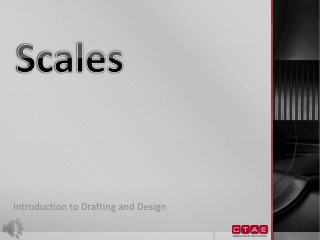DownloadDownload PresentationScales

# Scales

Télécharger la présentation## Scales

- - - - - - - - - - - - - - - - - - - - - - - - - - - E N D - - - - - - - - - - - - - - - - - - - - - - - - - - -
##### Presentation Transcript

1. Scales Introduction to Drafting and Design

2. What is a scale?

3. Scale • There are two different meanings for the word scale • The size to which an object is drawn • An instrument with a system of ordered marks at fixed intervals used as reference standards in measurements. • A scale establishes a proportion used in determining the dimensional relationship of an actual object to the representation of the same object on a drawing.

4. What are the different types of scales?

5. Types of Scales • There are four different shapes of scales: • Two Bevel • Four Bevel • Opposite Bevel • Triangular • Triangular shaped scales are the most common scale used.

6. Types of Scales • There are three major scales are used in today’s industries • Architect Scale • Engineer Scale • Metric Scale

7. Architect Scale • Used to make drawings of buildings and building detail • Six sided with 11 different scales • Common scales on an architect scale other than the “full” scale of 16 • The 16 or full scale is a standard ruler with each mark representing 1/16” of an inch • The scales are usually grouped together when one factor is twice the other • When scales are grouped together, one of the scales is read from one end of the instrument and the other scale is read from the opposite end.

8. Engineer Scale • Also known as a civil scale • Is used for measuring length and transferring length of larger scale plans such as site plans • An Engineer Scale is divided into 6 scales

9. Engineer Scale • The engineer scale and the architect scale are similar in appearance • The engineer scale is designed to be more precise by using a decimal scaling scheme • Whereas an architect’s scale uses fractional scaling. • Engineer scales are only read from left to right where architect scales can be read from left to right and right to left.

10. Metric Scale • Used for drawings made in metric units or meters • The metric scale uses ratios

11. How do we use each scale?

12. How do we use all of the scales? • To make a measurement, observe the scale from directly above. • Mark the desired measurement on the paper by using a light perpendicular line made with a sharp pencil • Keep you scale clean • DO NOT mark on the scale itself • DO NOT use the scale to draw straight lines

13. Architect Scale • To take a measurement with an architect’s scale follow these steps: • Find the scale that matches the scale of the drawing • Align the zero mark of the scale with one side of the object • Read the foot measurement by finding the closest tick mark on the scale that is to the end of the object without going past the end of the object. • Then read the inch measurement by counting the number of small tick marks that are located past the large tick mark.

15. Engineer Scale • To take a measurement with an engineer’s scale, first obtain the scale on the drawing. • The scale will either be in an inch to feet equality (1” = 20’) or a ratio (1:20) • Locate the matching scale on the engineer’s scale and line up the zero on the left side with the object being measured. • Find the tick mark that lines up with the other end. • Read the major marks and add the minor marks as the tenths and hundredths.

16. Examples Use the ratio 1” = 10’ Answer: 15.5’ Use the ratio 1” = 30’ Answer: 56’ Use the ratio 1” = 600’ Answer: 1150’

17. Metric Scale • Metric scales can be used to reduce or enlarge a drawing • Most metric drawings are drawn with a 1:1 metric scale. • To use a metric scale, find the correct ratio that matches the drawing ratio • Place the end of the object on the zero line • Find the large tick mark to indicate the tens, then use the little tick marks to find the ones and decimal numbers.